﻿ Properties of a Certain Class of Meromorphic Analytic Functions Defined by a Linear OperatorPublications are Open
Access in this journal
Article Versions
Export Article
• Normal Style
• MLA Style
• APA Style
• Chicago Style
Research Article
Open Access Peer-reviewed

### Properties of a Certain Class of Meromorphic Analytic Functions Defined by a Linear Operator

Ajai P.T. , Moses B.O., Ihedioha S.A.
American Journal of Applied Mathematics and Statistics. 2019, 7(5), 167-170. DOI: 10.12691/ajams-7-5-2
Received September 10, 2019; Revised October 12, 2019; Accepted October 25, 2019

### Abstract

In this present paper, we introduced and characterized a new class of meromorphic univalent functions associated with polylogarithm by investigating; coefficient inequality, convolutions property, integral means and other properties of the class.

### 1. Introduction and Definitions

Let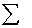denote the class of functions of the form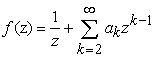(1.1)

Which are analytic in the unit disk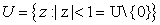. Having a simple pole at the origin with residue 1. Furthermore, let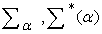and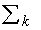,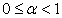denotes the subclasses ofwhich are univalent, meromorphically starlike and convex respectively.

Definition 1

Analytically, a function of the form (1.1) is in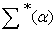if and only if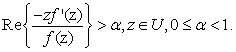(1.2)

Definition 2

Similarly,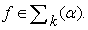If and only if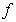is of the form (1.1) and satisfies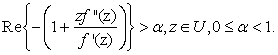(1.3)

Definition 3

For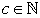, the set of natural numbers with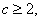an absolutely convergent series defined as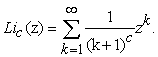(1.4)

Is known as the polylogarithm. This class of functions was invented by Liebniz and Bernouli 1. For more works on polylogarithm and meromorphic functions see 2, 3, 4, 5, 6, 7.

We state here a linear operator derived as follow;

Let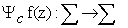which is defined by the following Hadamard product by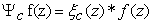Where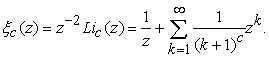(1.5)

Define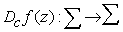as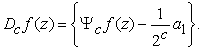(1.6)

Definition 4

Let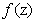be defined as in (1.1) and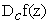as stated in (1.6) then the functionthen the functionin (1.1) is said to be in class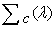if the following geometric condition are satisfy;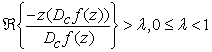(1.7)

Using subordination we write (1.7) as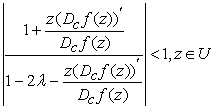(1.8)

Where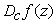is as defined in (1.6)

### 2. Coefficient Inequality

Theorem 2.1

Let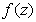of the form (1.1) a functionis said to be in the class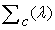iff the following bound is satisfy: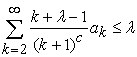(2.1)

Proof

Assume that (2.1) holds true then from (1.8) we have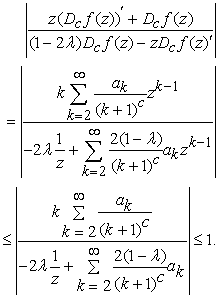Proving (2.1) Conversely, suppose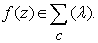We have to show that condition (2.1) is true. Thus we have(2.2)

Which is equivalent to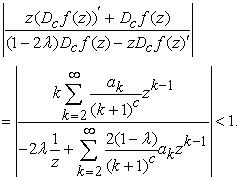Notice that since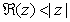we similarly have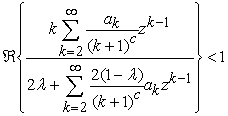(2.3)

We choose the value z on the real axis and letting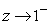, we have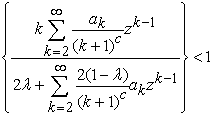(2.4)

Which proves our assertion. The result is sharp here for the function;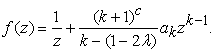(2.5)

Theorem 2.2

The class is closed under convex combination.

Let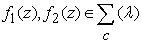then for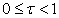, then we have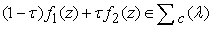.

Proof

By hypothesis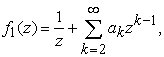and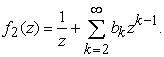Then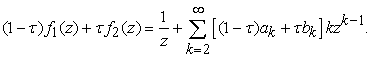Thus we have from (2.1) the following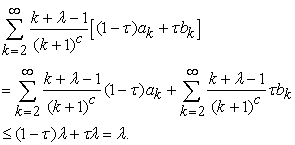This complete our proof.

### 3. Integral Means Inequalities

Letand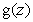be analytic in U,is said to be subordinate to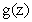written as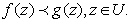(3.1)

If there exists a Schwarz function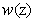which is analytic in U with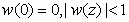,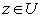such that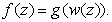Furthermore, if the function g(z) is univalent in U, then we have the following equivalence , see 8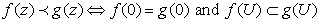.

Theorem 3.1 9

If f(g) and g(z) are analytic in U with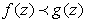, then for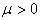, and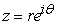,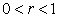. Then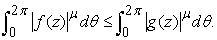Theorem 3.2

Let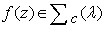and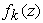be defined by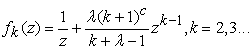if there exists w(z) such that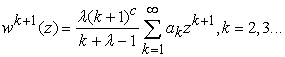(3.2)and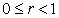. Then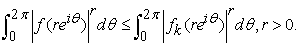Proof

It is obvious that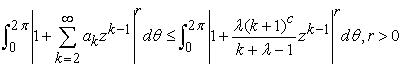Using theorem 3.1 we have to show that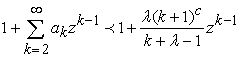(3.4)

Suppose we set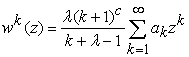. Then we have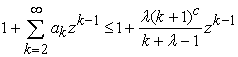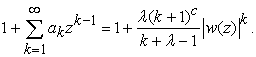Notice that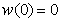and from theorem 2.1 we can write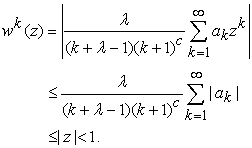This proves our theorem.

### 4. Convolution Property

Let,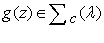and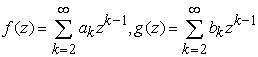Robbinson 10 has shown that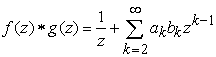is also in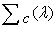.

Theorem 4.1

Suppose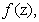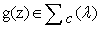then the Hadamard product or convolution of the functions f and g belongs to the class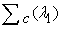. Where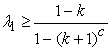.

Proof.

Since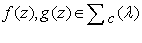, from theorem 2.1 we have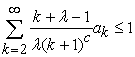and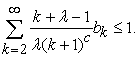We need to find the largest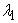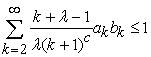, by Cauchy-Schwarz inequality, we have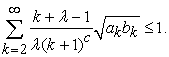(3.5)

Thus it suffices to show that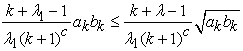Which is equivalent to

But from (3.5) we have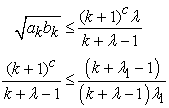The above simplify to. This proves our result.

### Acknowledgements

The authors are thankful to the referees for their valuable suggestions. The first Author appreciates the directorate of Technical Aids Corps (TAC) for the privilege accorded me to be deployed as volunteers to The Gambia.

### References

  Gerhardt C. I., Leibniz G.W, Mathematische Schriften III/1, Georg Olms, NY, USA, 1971. In article  Al-Shaqsi K. and Darus M , A multiplier transformation defined by convolution involving nth order polylogarithm functions, International Mathematical Forum, 4 (37), 1823-1837, 2009. In article  Al-Amiri H.S and Reade M.O, On linear combination of some expression in the theory of Univalent functions, Monatsh maths 80, 257-264, 1975. In article View Article  Bajpai S.K, A note on a class of meromorphic univalent functions, Rev. Rownanie Math. Pures Appl. 22, 295-2971977. In article  Goncharov A. B, Polylogarithms in arithmetic and geometry, Proceedings of the International Congress of Mathematicians, 374-387, Zurich, Switzerland, August 1994. In article View Article  Goel R.M. and S o h i N. S, on a class of meromorphic functions, Glasnik Matematioki 17 (1981), 19-28. In article  Rashhed K. A, Darus M., A new class of meromorphicfunctions involving polylogarithm function: Journal of complex analysis, (2014), 135-140. In article View Article  Miller S.S. Mocanu, P.T. Differential Subordinations.Theory and Applications,Series on Monographs and Textbooks in Pure and Appl. Math. No. 255, Marcel Dekker Inc.,New York, (2000). In article View Article  Littlewood J.E, On inequalities in the theory of functions, Proceedings of the London Mathematical Society, 23(1) 481-519, 1925. In article View Article  Robertson M.S, Convolutions of schlicht functions, Proc. Amer. Math. Soc. 13 (.1962), 585-589. In article View Article

Published with license by Science and Education Publishing, Copyright © 2019 Ajai P.T., Moses B.O. and Ihedioha S.A.This work is licensed under a Creative Commons Attribution 4.0 International License. To view a copy of this license, visit http://creativecommons.org/licenses/by/4.0/